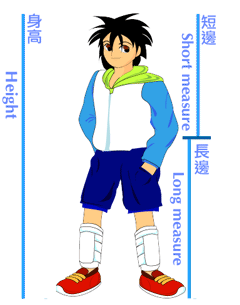t2 mx sc1 5.3 Statistics Φ Name:FLFiboBelly ratio
AS 1.600
YW 1.636
HP 1.655
CH 1.655
LR 1.690
LN 1.714
GS 1.714
AG 1.720

The FiboBelly ratio is the ratio of your height in centimeters divided by the height of your belly button (your height divided by the long measure in the diagram). A well circulated myth is that this ratio is, on average, the golden ratio Φ = $\frac{1+\sqrt{5}}{2}$ . Retain four face digits in your answers! Enter data carefully, double check your data entry!

1. __________ What level of measurement is the data?
2. __________ Calculate the sample size n for the data.
3. __________ Determine the minimum.
4. __________ Determine the maximum.
5. __________ Calculate the range.
6. __________ Calculate the midrange.
7. __________ Determine the mode.
8. __________ Determine the median.
9. __________ Calculate the sample mean x.
10. __________ Calculate the standard deviation sx.
11. __________ Calculate the sample coefficient of variation CV.
12. __________ If this data were to be divided into three classes, what would be the width of a single class?
13. Determine the frequency and calculate the relative frequency using three classes. Record your results in the table provided.
FiboBelly CUL (m)Frequency (f)Relative Frequency p(x)
Sum:
14. Sketch a frequency histogram chart of the data anywhere it fits, labeling your horizontal axis and vertical axis as appropriate.
15. ____________________ What is the shape of the distribution?
16. __________ Use the sample mean x and standard deviation sx calculated above to determine the z-score for Shanalin's fibobelly ratio of 2.036.
17. ____________________ Is the z-score for a fibobelly ratio 2.036 an ordinary or unusual z-score?
18. __________ What is the probabity that a fibobelly ratio is greater than 1.68?
19. __________ What is the probability of rolling a four or a multiple of four on a twelve sided die numbered one to twelve?
20. NameFiboBelly ratioHeight
Dana 1.61967
Marlin 1.72158.5
Tristan1.85037
The table contains fibobelly data verus height data for three individuals.
_________ Calculate the sample size n.
21. _________ Calculate the slope of the linear regression.
22. _________ Calculate the y-intercept of the linear regression.
23. _________ Is the relationship positive, negative, or neutral?
24. _________ Calculate the correlation coefficient r for the data.
25. ______________ Is the correlation none, weak/low, moderate, strong/high, or perfect?
26. _________ Determine the coefficient of determination.
27. _________ What percent in the variation in the fibobelly ratio "explains" the variation in height?
28. _________ Use the slope and intercept to predict the height for a fibobelly ratio of 1.800
29. _________ Use the slope and intercept to predict the fibobelly ratio for a height of 50 inches.

Note: The relationship above is not statistically significant - the sample size is far too small. Over a larger sample the variables relate randomly at a given age. For the purposes of this test the sample size was kept small.